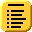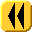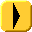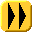## Chapter 15: Boundary-Value ProblemsIf a 2nd-order ordinary differential equation in y(t) is prescribed to have two fixed values y(a) = ya and y(b) = yb, then together they define a boundary-value problem (BVP).

In two dimensions we will examine elliptical partial differential equations (PDEs) with Dirichlet conditions. We will focus on a special case, that of Laplace's equation.

This topic looks at a single technique of finite differences which may be used to find solutions to such problems. The finite element method is described but not persued.